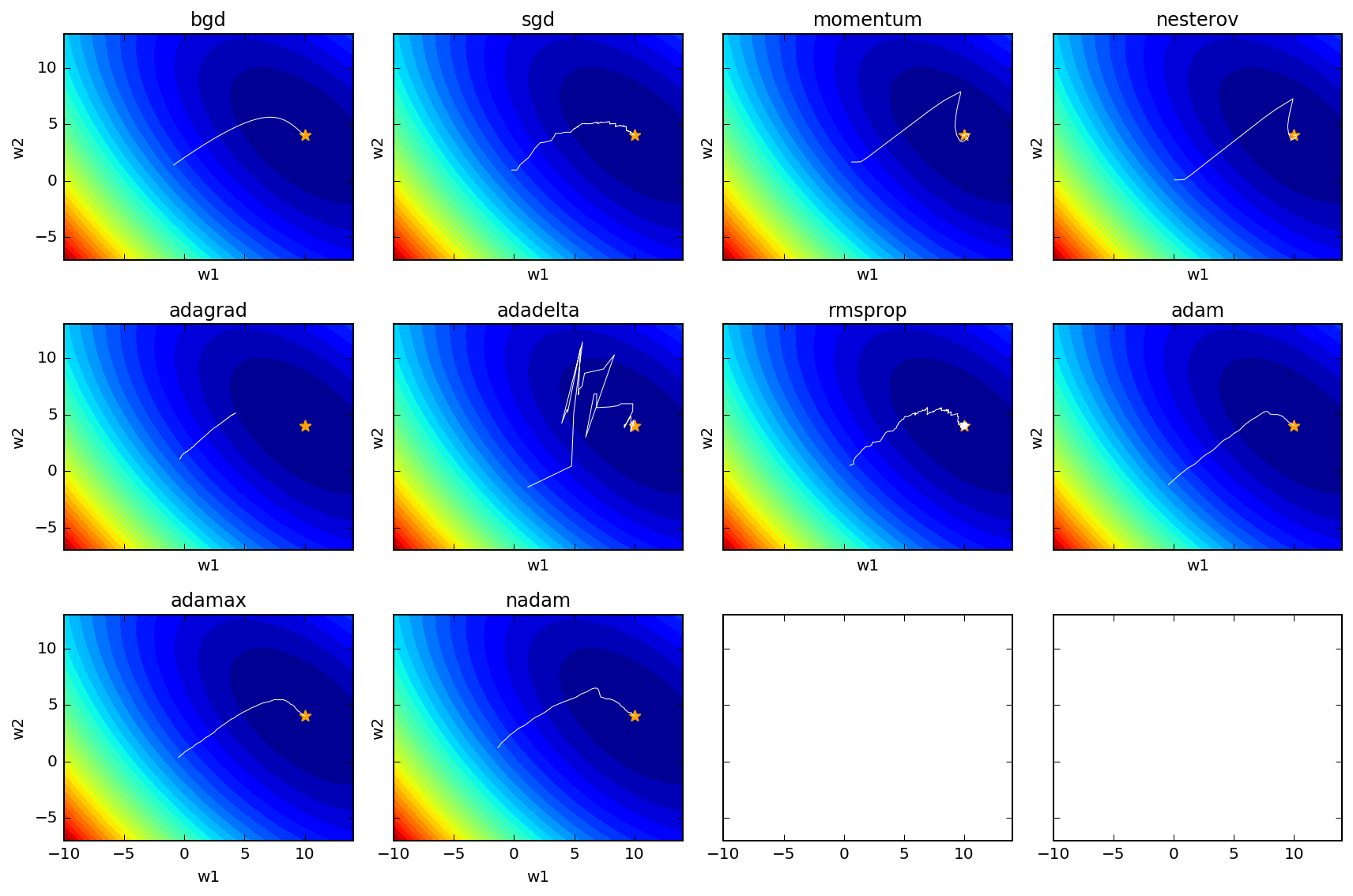Recently, I implemented multiple gradentient descent-related optimization algorithms and applied to a simple loss function for visualization purpose.

The result look like suchLegend:

• The contour shows the surface of the loss function given the sampled data and the model function, a quadratic function with two parameters (w1 and w2).
• The star indicates the true parameter of the quadratic function, from which the data is sampled.
• The white line is the trajectory of gradient descent with the corresponding algorithm.

• Batch gradient descent is the most smooth
• Stochastic gradient descent is more jumpy
• Plain momentum and Nesterov accelerated momentum (NAM) overshoot and then correct themselve
• Adagrad gets stuck as $G$ continues to increase adding squared gradients at each time step, effectively keeping reducing the learning rate to become infinitesimally small, thus learning gets halted.
• RMSprop is quite similar to Adagrad, replacing $RMS[\Delta \theta]_{t-1}$ with $\eta$, but it doesn’t seem to be as good as Adadelta based on the above loss function trajectory.